# NCERT Solutions for Class 12 MathsClass 12 NCERT Maths Solutions contains step-by-step answers to all 13 chapters. Students who are preparing for their CBSE Board exams, State Board Exams or competitive exams like JEE, can refer to these NCERT Solutions for Class 12 Maths.

These solutions allow students like you to understand the complex chapters such as Derivatives, Integrals, Application of Integrals, etc.

The complete solution is explained in an easy manner so that learners can solve chapter wise questions, examples, miscellaneous problems, and Intext Questions easily.

These solutions consist of error-free answers to all the questions given in NCERT Maths Textbooks.

If you want to understand the maths questions in an easy and quick manner then get Class 12 Maths NCERT Solutions for chapter-wise exercises, examples, miscellaneous problems, and Intext Questions.

The whole NCERT Solutions For Class 12 Maths are explained in an easy-to-understand manner. It helps in having a precise and step-by-step answer to the questions mentioned in the NCERT Class 12 Maths Textbook.

Follow the below-given tables to find out the class 12 maths NCERT solutions as per your requirement. It is free to download and can be accessed in a PDF file.

Because every question and their answers are explained very briefly so, it saves students time and energy to find out the correct steps of maths solutions.

It is considered a very helpful study material for standard XII students but that is not its limit.

Any students who are keen to prepare for the National level competitions or entrance exams such as JEE, BITSAT, VITEEE, etc, that are conducted every year can use the solutions that are provided herein chapter-wise, and exercise-wise.

These are provided by the Selfstudy’s maths experts. Hence, it is free and error-free too. You can download all these NCERT Maths Solutions for class 12th from this page.

## Class 12 Maths NCERT Solutions PDF for Chapter WiseTo excel and get good marks in the board exams students will have to prepare for the exams accordingly. For that reason, the NCERT Solutions For Class 12 Maths for all the chapters is given here.

It consists of problems and detailed solutions to give students immersive and smooth learning experiences.

Any student looking for a resource that can help them to boost their maths exam preparation can use the NCERT Solutions for Class 12 Maths PDF; Below given table contains the Solutions chapter-wise.

 NCERT Solutions Class 12 Maths Chapter Wise Chapter 1 - Relations and Functions Chapter 2 - Inverse Trigonometric Functions Chapter 3 - Matrices Chapter 4 - Determinants Chapter 5 - Continuity and Differentiability Chapter 6 - Applications of Derivatives Chapter 7 - Integrals Chapter 8 - Applications of Integrals Chapter 9 - Differential Equations Chapter 10 - Vector Algebra Chapter 11 - Three dimensional Geometry (3D) Chapter 12 - Linear Programming Chapter 13 - Probability

### NCERT Solutions for Class 12 Maths (Chapter 1 Relations and Functions)The first chapter of NCERT Class 12th Maths is relations and functions. It teaches the students about types of relations, types of functions, the composition of functions and invertible functions, binary operations along with miscellaneous examples.

Here, you can find the links for the topics covered in this chapter.

#### Most Important Formulas for NCERT Solutions Class 12 Maths Chapter 1 Relations and Functions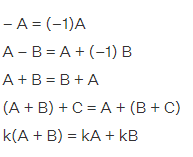where A, B and C are matrices.

Other study materials For Chapter 1 Relations and Functions

NCERT & Other study materials For Chapter 1 are given here. There are various study resources for students that can help them to grasp the knowledge of chapter 1 Relations and Functions.

### NCERT Solutions for Class 12 Maths (Chapter 2 Inverse Trigonometric Functions)Inverse trigonometry can be simply defined as the inverse functions of basic trigonometry functions. It is used to measure the angles of any trigonometry ratio. This is the chapter that will be used to examine the student's mathematical knowledge on the basis of finding angles.

#### Most Important Formulas for NCERT Class 12 Maths Solutions Chapter 2 Inverse Trigonometric Functions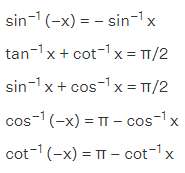What Topics Covered in Class 12 Maths Chapter 2 (Inverse Trigonometric Functions)?

Topics that are covered in Class 12 Maths NCERT Solutions (Inverse Trigonometric Functions) are as follows:- Basic Concepts of Trigonometric Functions, determining what is Domain, Detecting the value of inverse trigonometric functions at a specific point.

Also the methods to simplify the trigonometric expressions using the properties of inverse trigonometry are discussed in the second chapter of Class 12 maths.

This chapter has a total of 52 questions which vary in difficulty levels. There are 14 hard questions, 18 problems are at moderate level, & 20 questions are very easy to answer.

Other study materials For Chapter 2

NCERT & Other study materials For Chapter 2 are given here. There are various study resources for students that can help them to grasp the knowledge of chapter 2.

### NCERT Solutions for Class 12 Maths (Chapter 3 Matrices)Class 12th maths chapter 3 Matrices is one of the most important chapters for class 12th board exams. It is very simple and interesting to study. It is related to the calculations of matrices in the form of addition, subtractions, multiplications, etc. This chapter requires a very focused mind, in order to solve questions you will need to read them thoroughly and then give special attention while solving the questions.

However the solutions of matrices that we are giving here are very easy to understand, it will ease your process to comprehend the complex calculations.

#### Class 12 Maths NCERT Solutions Chapter 3 Matrices (Exercise: 3.1 - 3.4)

What Topics Covered in Class 12 Maths Solutions Chapter 3 (Matrices)?

The topics a student will find in Class 12 Maths NCERT Solutions (Chapter 3 Matrices) are as mentioned:- Intro to matrix, their types, properties, uses of equivalent matrices to find undisclosed quantities.

All these topics are extremely important to study and that is why chapter 3 has discussed related subtopics in detail.

One can find 78 questions in lesson 3 Matrices out of which barely 36 problems are tough to answer, the remaining 28 questions are at a moderate level and 14 questions are too easy to answer.

Other study materials For Chapter 3

NCERT & Other study materials For Chapter 3 are given here. There are various study resources for students that can help them to grasp the knowledge of chapter 3.

### NCERT Solutions for Class 12 Maths (Chapter 4 Determinants)NCERT Class 12th Maths Chapter 4 Determinants solutions aid in solving the given 4 exercises and the questions of this chapter. Determinants are a scalar value that is a function of the square matrix. In this lesson, you will get to know about the definitions and meaning of determinants, their properties, application of determinants, etc.

The Solutions that we are providing here for the Determinants are extremely helpful to solve the questions mentioned in the NCERT Books of Class 12.

#### Class 12 Maths NCERT Solutions Chapter 4 Determinants (Exercise: 4.1 - 4.6)

What Topics Covered in Class 12 Maths NCERT Solutions Chapter 4 (Determinants)?

The topics discussed in chapter 4 Determinants are brief on Determinants, various formats of determinants, their properties, Introduction to Minors and Cofactors and how to write them, etc.

Based on the topics and subtopics discussed in this lesson, 87 questions are asked in Class 12 Maths NCERT Chapter 4.

Out of those eighty-seven questions, only a total of 40 questions are tough and can be answered if one has a conceptual understanding of the topic.

Other study materials For Chapter 4

NCERT & Other study materials For Chapter 4 are given here. There are various study resources for students that can help them to grasp the knowledge of chapter 4.

### NCERT Solutions for Class 12 Maths (Chapter 5 Continuity and Differentiability)Chapter 5 of NCERT Maths Textbooks starts with the introduction of the topic and the definition of continuity and differentiability. This chapter is the continuous study of differentiation of function which was in class XI. In this chapter, students get to know about the algebra of continuous function, definition and meaning of differentiability, derivatives of composite functions, derivative of implicit functions, the derivative of inverse trigonometric functions, exponential and logarithmic functions, etc.

It has a few concepts that are very difficult to solve but our NCERT Solutions For Class 12 Maths Chapter 5 can help you to solve them very easily.

#### Important Formulas for NCERT Solutions Class 12 Maths Chapter 5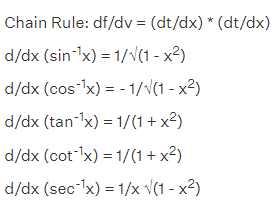What Topics Covered in Class 12 Maths NCERT Solutions Chapter 5 (Continuity and Differentiability)?

The whole topics in Class 12 Maths NCERT Solutions are distributed in various subtopics: Definition of continuity, algebra of continuous functions, Chain Rule theorem, Mean Value theorem, the derivation of implicit functions, etc. are covered in this chapter 5 Continuity and Differentiability.

Since this lesson has covered lots of topics, there are numerous questions. The NCERT 12 Maths Textbook Chapter 5 contains a total of 144 problems that vary on the basis of difficulty levels.

Other study materials For Chapter 5

NCERT & Other study materials For Chapter 5 are given here. There are various study resources for students that can help them to grasp the knowledge of chapter 5.

### NCERT Solutions for Class 12 Maths (Chapter 6 Applications of Derivatives)Chapter 6 Application of Derivatives introduces the learners to Finding the Rate of Change of a Quantity, Finding the Approximation Value, Finding the equation of a Tangent and Normal To a Curve, Finding Maxima and Minima, and Point of Inflection, Determining Increasing and Decreasing Functions, etc. In order to be well versed in these topics, students need to solve their questions. A few of them can be easily solved, but a few can be a little challenging to answer.

Therefore, the NCERT Solutions for Chapter 6 is available here that will aid in answering those questions properly.

#### Class 12 Maths NCERT Solutions Chapter 6 Application of Derivatives (Exercise: 6.1 - 6.5)

What Topics Covered in Class 12 Maths NCERT Solutions Chapter 6 (Applications of Derivatives)?

The topics that are available in Class 12 Maths NCERT Solutions chapter 6 are very interesting as well as linked to the previous lessons.

The topics and subtopics discussed in chapter 6 of Class 12 Maths are - Rate of Change of Quantities, uses of differentiation to determine whether a function is increasing or decreasing, etc. Students can refer to the CBSE Syllabus of Class 12 Maths to check the complete topics of chapter 6.

Referring to all the topics and subtopics available in chapter 6, the experts have developed 126 total questions which vary in difficulty level.

Other study materials For Chapter 6

NCERT & Other study materials For Chapter 6 are given here. There are various study resources for students that can help them to grasp the knowledge of chapter 6.

### NCERT Solutions for Class 12 Maths (Chapter 7 Integrals)Chapter 7 Integrals is a topic of calculus where you can see the definition of indefinite integral, integration as an inverse process of differentiation, geometrical interpretation of indefinite integral, properties of indefinite integral, comparison between differentiation and integration, methods of integration such as integration by substitution, integration using partial fractions, integration by parts, integration using trigonometric identities, etc.

We have provided the solutions of integrals for all the questions available in the mathematics book of class 12.

### Class 12 Maths NCERT Solutions Chapter 7 Integrals (Exercise: 7.1 - 7.8)

What Topics Covered in Class 12 Maths NCERT Solutions Chapter 7 (Integrals)?

To help students to unlock their potential of answering the maths questions chapter 7 Integrals has covered many topics: Inverse Process of Differentiation, Geometrical interpretation of indefinite integral, and many more.

The chapter has a total of 271 questions where 115 questions are very easy as they are based on formulas. Students can get complete step by step answers to each question in Class 12 Maths NCERT Solutions.

Other study materials For Chapter 7

1. Online MCQ Test Chapter 7 Integrals
2. MCQs PDF Chapter 7 Integrals
3. NCERT Books For Chapter 7
4. NCERT Exemplar Solutions For Chapter 7
5. NCERT Exemplar Books For Chapter 7
6. Revision Notes For Chapter 7
7. RD Sharma Solutions For Chapter 7 (Indefinite & Definite)
8. RS Aggarwal Solutions For Chapter 7 (Indefinite & Definite)
9. HOTS Questions For Chapter 7

### NCERT Solutions for Class 12 Maths (Chapter 8 Applications of Integrals)Chapter 8 of NCERT Class 8 maths textbook is Applications of Integrals. This chapter aids in knowing how to calculate the areas under simple curves, the area between lines, parabolas, etc. It can also be used to calculate the area of an enclosed figure. However, in order to do the above-given things, you have to know the Application of Integrals. To do so, practising the questions is ideal. Also, it plays a crucial role in class 12th mathematics examination so solve these questions as much as possible.

#### Class 12 Maths NCERT Solutions Chapter 8 Application of Integrals (Exercise: 8.1 - 8.2)

What Topics Covered in Class 12 Maths Solutions Chapter 8 (Application of Integrals)?

The chapter 8 Application of Integrals has several topics which allow learners to learn the methods of using the Integrals to find areas. Area between two curves, 2 Area under Simple Curves, etc. are covered in this lesson.

Based on these topics about 39 questions are solved in Class 12 Maths NCERT Solutions.

Other study materials For Chapter 8

NCERT & Other study materials For Chapter 8 are given here. There are various study resources for students that can help them to grasp the knowledge of chapter 8.

### NCERT Solutions for Class 12 Maths (Chapter 9 Differential Equations)Chapter 9 Differential Equations is a mathematical equation that relates to one or more functions and their derivatives. Differential equations are considered special because the solution of a differential equation is itself a function instead of a number. In this chapter, you will be able to study the basic concepts related to differential equations, the degree of a differential equation, the order of a differential equation, and many more.

The solutions for this chapter have been given here to help you out while getting stuck on any question of differential equations in chapter 9 of Class 12 NCERT Maths.

#### Class 12 Maths NCERT Solutions Chapter 9 Differential Equations (Exercise: 9.1 - 9.6)

What Topics Covered in Class 12 Maths NCERT Solutions Chapter 9 (Differential Equations)?

Lesson 9 of class 12th maths covers all the relevant topics of Differential Equations from basics to intermediate level; such as - Order of a differential equation, degree of differential equations, also the method to form the differential equations when General Solutions are given.

A total of 113 problems are asked in this chapter that holds a good weightage in the examinations. Our experts have answered all those 113 problems in Class 12 Maths NCERT Solutions.

Other study materials For Chapter 9

NCERT & Other study materials For Chapter 9 are given here. There are various study resources for students that can help them to grasp the knowledge of chapter 9.

### NCERT Solutions for  Class 12 Maths (Chapter 10 Vector Algebra)Vector Algebra is the 10th chapter of NCERT Class 12th Mathematics textbook. This chapter introduces the students to the algebra of vector quantities. It is an essential topic of mathematics, it also impacts the study of Physics. So students must focus on this chapter and solve their questions to get a good grasp on this topic.

The given solutions will acquaint you with this topic in detail with solutions.

#### Class 12 Maths NCERT Solutions Chapter 10 Vector Algebra (Exercise: 10.1 - 10.4)

What Topics Covered in Class 12 Maths NCERT Solutions Chapter 10 (Vector Algebra)?

Topics that are covered under Class 12 Maths NCERT Solutions are an introduction to Vector algebra, their types, several methods to perform vector algebra addition, subtraction, multiplications and more.

A total of 63 problems are given out of which 20 problems require less effort to solve.

Other study materials For Chapter 10

NCERT & Other study materials For Chapter 10 are given here. There are various study resources for students that can help them to grasp the knowledge of chapter 10.

### NCERT Solutions for Class 12 Maths (Chapter 11 Three Dimensional Geometry - 3D)Chapter 11 Three Dimensional Geometry helps the students to study the direction cosines and direction ratios of a line joining two points, it also discusses the equations of lines and planes in space under different conditions, the angle between two lines, two planes, a line and a plane, the shortest distance between two skew lines and distance of a point from a plane, etc.

3D has various applications or implementations in the real world and the field of mathematics. Therefore, we have given the NCERT Solutions for 3D. It has a very precise or to-the-point answer. All are explained in detail.

#### Important Formulas for NCERT Solutions Class 12 Maths Chapter 11where a1, b1, c1 and a2, b2, c2 are direction ratios and θ is the acute angle between the two lines.

What Topics Covered in Class 12 Maths Solutions Chapter 11 (3D - Three Dimensional Geometry)?

This whole lesson discussed various topics based on the direction; such as Relation between the direction cosines of a line, What is Direction line, and Cosine, etc.

This chapter has 59 questions out of which only 12 questions are complex to answer. However, by using the Class 12 Maths NCERT Solutions all these questions are easily answered.

Other study materials For Chapter 11

NCERT & Other study materials For Chapter 11 are given here. There are various study resources for students that can help them to grasp the knowledge of chapter 11.

### NCERT Solutions for Class 12 Maths (Chapter 12 Linear Programming)In this chapter, students get to know about Linear programming. Linear Programming is also known as L.P is the simplest way to solve complex optimizations in a simple manner. The complex relationship can be depicted through linear programming, and chapter 12 is all about these things. It helps you to understand how to simplify the relationship in simple terms.

Here on this website, we have given the Linear Programming Solutions for all exercises. It is errorless and available free of cost.

#### Class 12 Maths NCERT Solutions Chapter 12 Linear Programming (Exercise: 12.1 - 12.2)

What Topics Covered in Class 12 Maths Solutions Chapter 12 (Linear Programming)?

The mathematical formulation of the problem, Graphical method of solving linear programming problems, various theorems are given in class 12 maths chapter 12 Linear Programming.

There are a set of 31 questions that are distributed among various exercises. About 14 questions are very simple to answer out of the given 31 problems.

To solve all those 31 questions, Class 12 Maths NCERT Solutions can be a great study resource.

Other study materials For Chapter 12

NCERT & Other study materials For Chapter 12 are given here. There are various study resources for students that can help them to grasp the knowledge of chapter 12.

### NCERT Solutions for Class 12 Maths (Chapter 13 Probability)The probability chapter of class 12th is a little advancement from the class 11th probability lesson. In this chapter, you will be using the information you have acquired in class 11th such as conditional probability, properties of conditional probability, multiplication theorem on probability, multiplication rule of probability for more than two events, independent events, Baye’s theorem, etc.

We have compiled here the solutions to exercises from the Probability chapter, which have explained the concept thoroughly.

#### Class 12 Maths NCERT Solutions Chapter 13 Probability (Exercise: 13.1 - 13.5)

What Topics Covered in Class 12 Maths NCERT Solutions Chapter 13 (Probability)?

The most important and widely used topic: conditional probability is a part of Class 12 Maths NCERT Solutions Chapter 13 Probability.

Their types as well as Multiplication Theorem on Probability, Baye’s Theorem, etc. have been covered in this lesson.

100 Questions are asked out of which 23 are pretty simple problems to solve.

Other study materials For Chapter 13

NCERT & Other study materials For Chapter 13 are given here. There are various study resources for students that can help them to grasp the knowledge of chapter 13.

NCERT Solutions

We hope you have learned something from the provided solutions. You can download NCERT Solutions here by clicking on the given links. These solutions are free of cost.

### Chapter Wise Preparation Tips For Class 12 Maths [All Chapters]

#### Chapter 1:- Relations and Functions

The very first chapter of NCERT Solutions for Class 12 Maths is Relations and Functions. The relation indicates the relationship between INPUT and OUTPUT and It is known as a subset of the Cartesian product. While, function is a relation which presents one OUTPUT for each given INPUT.

To understand the Function properly we can take a look at the below given example:-

Every A-value should be associated with only one B-value. You can say here: A is a domain whilst B is a range. Note that, Domain and Range will not be repeated while writing them.

This topic may look very simple but the majority of students find it difficult to comprehend.

Both relations and functions have different types. Relations topics have types of reflexive, symmetric, transitive and equivalence, where the functions have One to one and onto, composite, the inverse of a function, etc.

1.1 Introduction: The intro section helps students to know how much they are aware of the topic Relations and Functions since it has been already covered in Class 11th Maths. Apart from this, the introduction part of this chapter tells what students will be able to study further in this lesson. There are various topics that will be covered in this lesson: different types of relations and functions, composition of functions, invertible functions and binary operations.

1.2 Types of Relations: After the overview of the lesson, the section 1.2 talks about different types of Relations:Empty Relation, Universal Relation, Identity Relation, Inverse Relation, Reflexive Relation, Symmetric Relation, Transitive Relation, Equivalence Relation.

1.3 Types of Functions:-In Class 11th students have already studied the special functions like identity function, constant
function, polynomial function, modulus function, signum function etc. as well as Addition, subtraction, multiplication and division of two functions have been covered too. But in this section 1.3 different types of functions have been covered. The One – one function (Injective function), Many – one function, Onto – function (Surjective Function), Into – function, etc. have been covered.

1.4 Composition of Functions and Invertible Function:- The section 1.4 of NCERT Class 12 Maths Chapter 1 covers the Composition of functions and the inverse of a bijective function. With some suitable examples this topic has been elaborated in detail. As well as various theorems have been covered.

1.5 Binary Operations:- Binary means two and students are already taught in their earlier classes how to do binary operations such as Addition, Subtraction, Multiplications, etc. However in this section 1.5 the binary operations on sets have been covered. About 14 definitions are given in this section 1.5 Binary Operations.

Study Tip for Relations and Functions:- You must start with the definitions of Relations & Functions because once it is clear to you, you will be able to comprehend the topic. To make this chapter easier for you, go through the introduction section of this lesson and read them thoroughly.

Make sure you have a good command over the types of Relations and Functions. Solve some given examples to clear your confusion.

#### Chapter 2:- Inverse Trigonometric Functions

NCERT Solutions for Class 12 Maths Chapter 2 Inverse Trigonometric Functions will help you to solve all the questions of Chapter 2. However, you must be thorough with the lessons by studying their Definition, range, domain, principal value branch, etc.

These are important to learn because this lesson discusses the inverse of trigonometry which is a further topic of basic trigonometry you have studied in the previous classes. Inverse Trigonometry functions are also known as Arcus functions, anti trigonometric functions or cyclometric functions.

Knowledge of this topic helps you to get the angle with any of the trigonometry ratios.

Important Formulas:-

The basic inverse trigonometric formulas are as follows:

 Inverse Trigonometric Functions Formulas Arcsine sin-1(-x) = -sin-1(x), x ∈ [-1, 1] Arccosine cos-1(-x) = π -cos-1(x), x ∈ [-1, 1] Arctangent tan-1(-x) = -tan-1(x), x ∈ R Arccotangent cot-1(-x) = π – cot-1(x), x ∈ R Arcsecant sec-1(-x) = π -sec-1(x), |x| ≥ 1 Arccosecant cosec-1(-x) = -cosec-1(x), |x| ≥ 1

2.1 Introduction:- Continuing the previous lesson’s topics,  this section 2.1 introduces restrictions on domains and ranges of trigonometric functions. As well as some elementary properties will also be discussed here in section 2.1.

2.2 Basic Concepts:- This section recalls the  trigonometric functions and their basic concepts. one-one and onto functions, principal value branch, etc. have been discussed in section 2.2 Basic Concepts.

2.3 Properties of Inverse Trigonometric Functions:- Here the section 2.2 of NCERT Class 12 Maths Chapter 2 gives students a glimpse of Properties of Inverse Trigonometric Functions, also the properties are proved in this section. This topic has been covered only on the basic level since the discussion for values of x for which inverse trigonometric functions are defined goes beyond the scope of the textbook.

Study Tip for Inverse Trigonometric Functions:- Revise your basics of Trigonometry before starting this chapter. Trigonometric functions are especially applicable to the right angle triangle, so you must have a good understanding of the right angle triangle to solve the problems given in the chapter 2 of Class 12 Maths.

#### Chapter 3:- Matrices

NCERT Maths of Class 12, Chapter 3 Matrices is quite an interesting topic. In this lesson, you will begin with the definition of Matrix Operations or Matrices.

Matrices are an array of real or complex numbers within a square shape, the numbers are represented in two ways Horizontally and Vertically. The horizontal line is known as raw and vertical lines are called columns.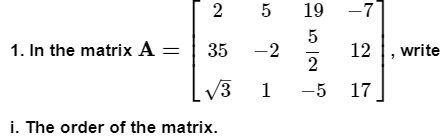In the above image, there is a question which contains one square matrix containing the real numbers as an element horizontally and vertically. The question wants you to ask the order of the matrix.

So, to answer this question, count the number of rows and columns, you will find it is in m×n, so the answer will be 3 × 4. The m represents here the number of rows where, n denotes the number of columns.

To solve the given exercise of chapter 3 Matix, make sure you have a good command on the below given

important formulas:

– A = (–1)A

A – B = A + (–1) B

A + B = B + A

(A + B) + C = A + (B + C)

k(A + B) = kA + kB

where A, B and C are matrices.

3.1 Introduction:- The intro part contains where matrices are used and how it can impact science and engineering whereas the section 3.1 also gives a brief what to study in this lesson such as fundamentals of matrix and matrix algebra.

3.2 Matrix:- This section introduces the term matrix and how to represent them in rows and columns. Along with the definition the order of matrices has been covered in subsection 3.2.1.

3.3 Types of Matrices:- There are various types of matrices: Column matrix, Row matrix, Square Matrix, Diagonal Matrix, Scalar Matrix, Identity Matrix, Zero Matrix. All of these types of matrices are defined in this section as well the equality of matrices are introduced in section 3.3.

3.4 Operations on Matrices:- Addition of matrices, multiplication of a matrix by a scalar, difference and multiplication of matrices are covered in the section 3.4. Along with these the properties of various Operations on Matrices are explained. The section 3.4 contains a total of 6 subsections to cover the whole operations on matrices.

3.5. Transpose of a Matrix:- When rows and columns are interchange in a matrix it is known as Transpose of a matrix. This section covers the transpose of a matrix and special types of matrices such as Symmetric and Skew Symmetric. In subsection 3.5.1 the properties of transpose of the matrices have been elaborated.

3.6 Symmetric and Skew Symmetric Matrices:- This section contains definitions of symmetric and skew symmetric matrices as well as the theorems have been proved too. After elaborating the topics and theorems a few examples are given to deepen the knowledge of Symmetric and Skew Symmetric matrices and its properties.

3.7 Elementary Operation (Transformation) of a Matrix:- Matrix has a total of 6 operations (Transformation) out of which three are due to rows and remaining 3 are due columns.

3.8 Invertible Matrices:- When two Square matrices such as Matrix A and Matrix B are equal such that AB=BA, then the B is called the inverse matrix of A and it is denoted by A– 1. Along with this definition the section 3.8 contains a few theorems and discussion of Inverse of a matrix by elementary operations.

Study Tip for Matrices:- This chapter is one of the lengthy lessons of NCERT Class 12 Maths so be patient and study all the given topics one by one. Understand all the concepts and keep revising the formulas from time to time.

#### Chapter 4:- Determinants

NCERT Class 12 Maths Chapter 4 introduces the topic determinants which is a scalar value. The given lesson elaborates the concept of the determinant of a square matrix and the ways to find it.

Below given image is an example of how determinants are represented. Determinant of A is denoted by |A| (or det A) and it is noted that only the square matrix can be a determinant.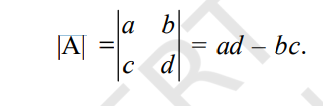To be able to understand this lesson and to solve the available questions, students should have a good understanding in properties of determinants, minors, cofactors, the inverse of a matrix, and linear equations.

4.1 Introduction:- This section tells learners what they are about to study in chapter 4 Determinants. According to section 4.1 this chapter deals with determinants up to order three with real entries. As well as various properties of determinants, such as minors, cofactors and applications of determinants in finding the area of a triangle, adjoint and inverse of a square matrix, etc. have been covered.

4.2 Determinant: What is determinant? The section 4.2 gives a definition of Determinant in section 4.2 as well as Determinant of a matrix of order one, order two, order 3 × 3 have been covered.

4.3 Properties of Determinants: Various properties of determinants have been covered in this section 4.3 which helps learners to simplify the evaluation process.

4.4 Area of a Triangle: The section 4.4 teaches students how to write the area of a triangle in determinant and how to calculate their value.

4.5 Minors and Cofactors: Minors and Cofactors help us to write the expansion of determinants in a compact manner. Therefore, section 4.5 helps students to know the definition as well as how to use these two terms to simplify the determinant's expansion.

4.6 Adjoint and Inverse of a Matrix: The condition for existence of inverse of a matrix as well as various theorems have been discussed in section 4.6 Adjoint and Inverse of a Matrix.

4.7 Applications of Determinants and Matrices: To check the system of linear equations in two or three variables as well as for checking the consistency of the system of linear equations have been covered in section 4.7.

Study Tip for Determinants:- Gain good command on square matrix and the concepts that are explained in the chapter’s beginning. Apart from this, you can take help of the NCERT Class 12 Maths Solutions.

#### Chapter 5:- Continuity and Differentiability

In NCERT Chapter 5, there are two different but related terms you have to study: Continuity and Differentiability. When Continuity of a function forms a continuous wave, it is called continuity. With such a function, one can draw a graph without lifting the pen.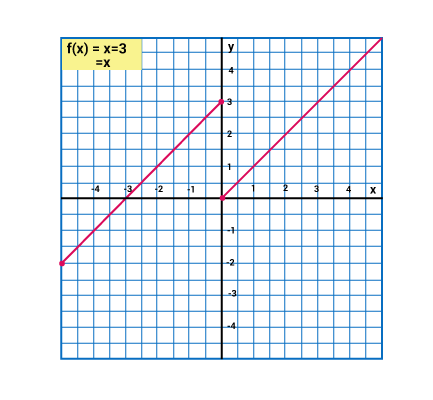In case the function is undefined it is known as discontinuous function.

It is believed by several students that Differentiability of functional parameters is quite difficult to learn.

5.1 Introduction: The intro section informs students what they can expect to learn in chapter 5. The chapter contains continuity, differentiability and relations between them. Inverse trigonometric functions as well as exponential and logarithmic functions have been covered too. The intro part is a must check section for all students as they can get some clue what to expect from Chapter 5 Continuity and Differentiability.

5.2 Continuity: The section 5.2 introduces students the term continuity as well as the definition of this term has been mentioned too. Apart from this, the important aspect of this section is to help students to know how to check the continuity of a given function.

5.3. Differentiability: This section has been divided into various subsections that help students to learn the topic in detail. Those various subsections are: (5.3.1) Derivatives of composite functions, (5.3.2) Derivatives of implicit functions, (5.3.3) Derivatives of inverse trigonometric functions.

5.4 Exponential and Logarithmic Functions: The definition number 3 says, the exponential function with positive base b > 1 is the function
y = f (x) = bx . Not only this definition, but there are more definitions available in section 5.4.

5.5. Logarithmic Differentiation: The section 5.5 helps students to learn to differentiate certain special classes of functions given in the form y = f (x) = [u(x)]v (x). The chain rule has been used in this section too.

5.6 Derivatives of Functions in Parametric Forms: This section 5.6 helps learners to know what parametric forms are and how to find Derivatives of Functions in Parametric Forms.

5.7 Second Order Derivative: The definition of second order derivatives, its notation and how to find second order derivatives are explained in the section 5.7.

5.8 Mean Value Theorem: Rolle’s Theorem and Mean Value Theorem are eleboarted in this section. Also, the geometric interpretation of these theorems have been discussed in section 5.8

Study Tip for Continuity and Differentiability:- You may find it difficult to comprehend but with the help of Class 12 Maths NCERT Solutions, you can answer all the questions with ease as well as you can access the step wise answer of all given questions. Apart from all these things, pay close attention to the important formulas:-

• Chain Rule: df/dv = (dt/dx) * (dt/dx)
• d/dx (sin-1x) = 1/√(1 - x2)
• d/dx (cos-1x) = - 1/√(1 - x2)
• d/dx (tan-1x) = 1/(1 + x2)
• d/dx (cot-1x) = 1/(1 + x2)
• d/dx (sec-1x) = 1/x √(1 - x2)

#### Chapter 6:- Applications of Derivatives

Chapter 6 of NCERT Class 12 Maths is going to be a very interesting chapter because this lesson helps students to learn how derivatives are used in real life.

Actually, the rate of change of one quantity with respect to another is known as derivatives. dy/dx = f(x) = y’ is defined in terms of a function. One can find the implication of this topic in various fields such as Science, Engineering, and other related fields.

Mainly derivatives are used to calculate the highest and lowest point of the curve in a graph, but one can also know the turning point of it.

6.1 Introduction: This section is dedicated to inform students what they have studied in the previous lesson and how it will be used in this lesson. The topics that were described in the previous chapter was finding derivatives of composite functions, inverse trigonometric functions, implicit functions, exponential functions and logarithmic functions. However, in this lesson applications of the derivative in various disciplines such as engineering, science, social studies and various other relevant fields have been discussed.

6.2 Rate of Change of Quantities: With the help of suitable examples the rate of change of quantities has been taught in this section.

6.3 Increasing and Decreasing Functions: The method of finding the increasing or decreasing function has been discussed in section 6.3.

6.4 Tangents and Normals:- This section helps students to understand how to find the equation of the tangent line and the normal line to a curve at a given point.

6.5 Approximations: The section helps students to learn the  use of differentials to approximate values of certain quantities.

6.6 Maxima and Minima: This section guide students to use the concepts of derivatives to calculate the maximum or
minimum values of various functions. Also, finding the turning point of a graph will be taught in this section 6.6.

Study Tip for Applications of Derivatives:- Be curious to know how you can implement the learning from this chapter into real life. It will help you to take interest in this subject more and more as well as doing so will assist you to score higher in the board examination.

#### Chapter 7:- Integrals

Integrals is the part of the calculus which is very important from the exam perspective. As per the definition, Integral is the representation of the area of a region under a curve. It is used to find various quantities such as areas, volumes, displacement, etc.

There are two types of integrals: Definite and Indefinite. The below given image contains a simple yet precise presentation of indefinite integrals.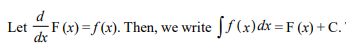One must have a good understanding of these two types of integrals to score fantastic marks in Class 12 Board Exam.

The various parts of this lesson includes integrals properties, limit, fundamental theorem of calculus, etc.

7.1 Introduction: Indefinite integral, definite integrals, Fundamental Theorem of Calculus, elementary properties including some techniques of integration have been covered in this lesson, and the introduction section talks about these things a bit.

7.2 Integration as an Inverse Process of Differentiation: Integration is known as the inverse process of differentiation. Instead of differentiating a function, a derivative of a function is given and asked to find its original function. This process is known as the integration. And so, this section 7.2 discusses the topic anti differentiation.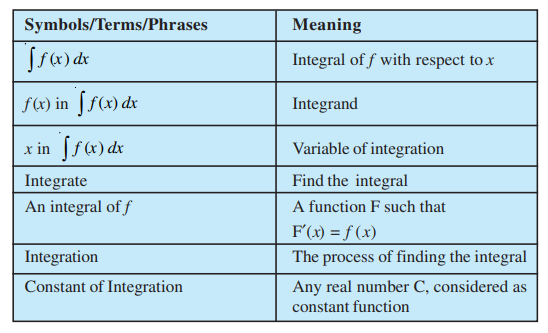Formulae for the Derivatives:7.3 Methods of Integration: Before reaching this section students were using the inception method to find the integrals. However, section 7.3 helps students to learn additional techniques for finding the integrals by reducing them into standard forms. The additional Methods of integration are based on:
1. Integration by Substitution
2. Integration using Partial Fractions
3. Integration by Parts

7.4 Integrals of Some Particular Functions: This section covers some important formulae of integrals and the methods of using them to integrate related standard integrals.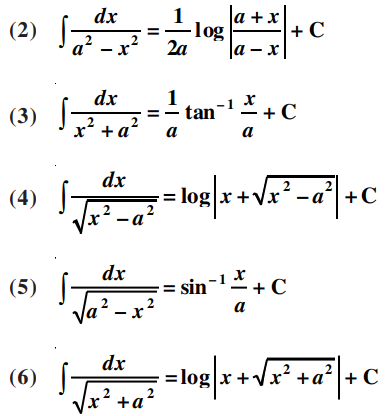7.5 Integration by Partial Fractions: Recalling the studied terms: rational function is defined as the ratio of two polynomials; this section has covered some of the related important formulas as well as a few examples. The section is dedicated to teach students how to do integration by partial fractions.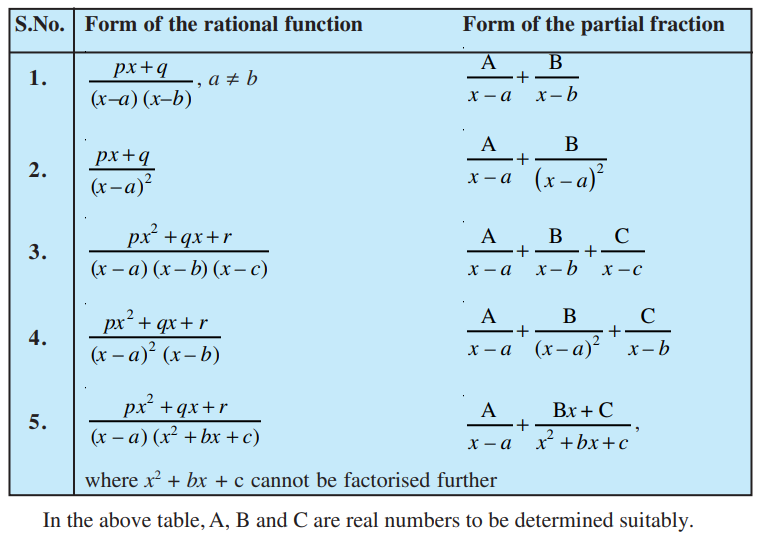7.6 Integration by Parts:- In addition to other methods of integration, this section deals with integration by parts. This method of integration is useful for integrating products of functions.

7.7 Definite Integral: This section is a kind of intro to definite integrals where the discussion revolves around what is Definite Integral and Definite integral as the limit of a sum.

7.8 Fundamental Theorem of Calculus: This section has covered a lot of important topics such as Area function, First fundamental theorem of integral calculus, Second fundamental theorem of integral calculus.

7.9 Evaluation of Definite Integrals by Substitution: Among lots of methods to find integration the most important methods of integration is by substitution. And so, this section 7.9 is dedicated for Evaluation of Definite Integrals by Substitution.

7.10 Some Properties of Definite Integrals: The section 7.10 talks about Some Properties of Definite Integrals. There are some formulae in this section that help students in evaluating the definite integral.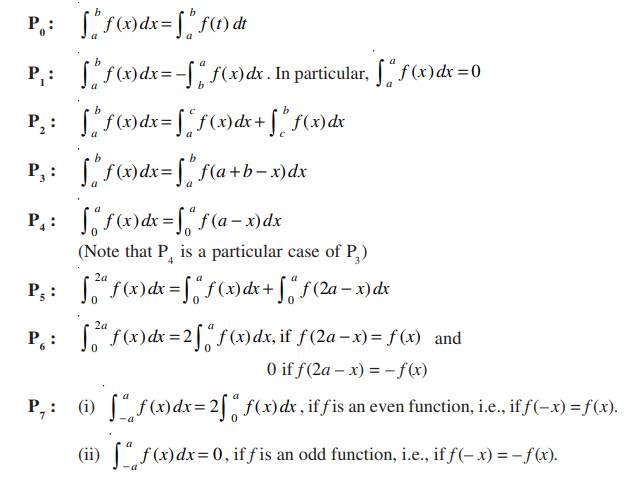Study Tip for Integrals:- This chapter is available in the PART 2 of NCERT Class 12 Maths Textbook and thus, the topics studied further will be a bit vast and complex. Hence, in the study tip, you are advised to maintain regularity and solve the same chapter multiple times. Integrals consist of Basic properties of definite integrals, fundamental theorems, etc. Developing a good grasp on these topics are essential and advised here too.

#### Chapter 8:- Applications of the Integrals

This chapter gives students a precise and comprehensive awareness of calculus as they can learn here how to find areas under the curve, between a curve and a line segment. In other words, NCERT Class 12 Maths Solutions Chapter 8 will help you to learn how to do the practical use of integrals.

8.1 Introduction: The intro section explains how the formulas to calculate areas of various geometrical figures that we have studied in earlier classes are ideal for simple figures only but, for areas enclosed by curves the same fundamental formula is not enough. For finding the areas enclosed by curves we need to have a proper knowledge of the Integral Calculus. The lesson 8 of NCERT Class 12 Maths covers this in detail.

8.2 Area under Simple Curves: This section is a part of finding the areas of an enclosed figure. This section covers the topic of the elementary area and areas under simple curves.

8.3 Area between Two Curves: Leibnitz integration has been introduced in section 8.3. Along with this the methods of finding the area between two curves have been mentioned.

Study Tip for Applications of the Integrals:- Keep in mind the learnings of Chapter 7 Integrals, as you may need to use the basics of that chapter to solve questions of Applications of the Integrals.

#### Chapter 9:- Differential Equations

An equation with a function of one or more of its derivatives is called Differential Equations. As per the definition the differential equation can be present like this,

dy/dx = f(x)

Here “x” is an independent variable and “y” is a dependent variable.

The differential equation is widely used in the field of Engineering, Biology, Physics, and Etc. One must be well versed with the previous chapter before starting to answer the questions asked in Class 12 Maths chapter 9.

9.1 Introduction:  Some basic concepts related to differential equations, general and particular solutions of a differential equation, formation of differential equations, three methods to solve a first order - first degree differential equation and some applications of differential equations in different areas have been covered in this chapter. The intro section helps students to know what topics they are going to study in NCERT Class 12 Maths Chapter 9.

9.2 Basic Concepts: The section 9.2 covers the concept of variables as well as derivatives of the dependent variable y with respect to the independent variable x. Such an equation is known as the Differential Equation and so their basic concepts have been covered here in this section.

9.3. General and Particular Solutions of a Differential Equation: General Solution is known as when the solution contains arbitrary constants. The solution free from arbitrary constants is called Particular Solutions. Refer to this section to study the terms in a proper manner.

9.4 Formation of a Differential Equation whose General Solution is given: This section deals with formation of a differential equation whose general solution is given. Along with this, the Procedure to form a differential equation that will represent a given family of curves is covered in section 9.4.

9.5. Methods of Solving First Order, First Degree: Differential Equations: In this section, students will be able to learn three methods of solving first order first degree differential equations. This section is distributed in various subtopics: (9.5.1) Differential equations with variables separable, (9.5.2) Homogeneous differential equations, (9.5.3) Linear differential equations.

Study Tip for Differential Equations:- Regular Practice, Daily revision of formulas and making notes are advised. This lesson should not be difficult for those who have good grasp over the previous lessons.

#### Chapter 10:- Vector Algebra

The knowledge of vectors is used in the field of Mathematics and Physics. As per the definition of vector, it has direction and magnitude. Vector is usually represented by an arrow (→) which shows the direction and its length shows the magnitude. It is denoted like this .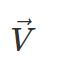In Class 12 Maths chapter 10, Zero Vector, Unit Vector, Coinitial Vectors, Collinear Vectors, and such more subtopics are explained.

The exercises given in this chapter help students to learn how to perform dot and cross products, section formulas, computing the magnitude of vectors, etc.

10.1 Introduction:  Some basic concepts about vectors, various operations on vectors, and their algebraic and geometric properties have been covered in this chapter. And so the introduction section allows students to get a brief on what they are about to study in this chapter.

10.2 Some Basic Concepts: The section 10.2 helps students to know some basic concepts of vectors that are initial point, terminal point, magnitude, Position Vector, Direction Cosines.

10.3 Types of Vectors: Section 10.3 allows a learner to learn about the types of vectors. There are various types of vectors and this section covers the following: Zero Vector, Unit Vector, Coinitial Vectors, Collinear Vectors, Equal Vectors, Negative of a Vector.

10.4 Addition of Vectors: Triangle law of vector addition, Properties of vector addition have been covered in this section.

10.5 Multiplication of a Vector by a Scalar: This section is dedicated to teach students how to multiply a vector and scalar vector. Components of a vector, Vector joining two points, Section Formula have been discussed here in this section. These topics have been covered in detail inside the NCERT Class 12 Maths Chapter 10.

10.6 Product of Two Vectors: Scalar (or dot) product of two vectors, Projection of a vector on a line, Vector (or cross) product of two vectors are covered here in this section. The study of these topics are important as it helps students to understand the Vector topic in detail.

Study Tip for Vector Algebra:- Commutative Law & Associative Law are two main laws of vector addition, so understand them as well as cover the introduction part by yourself before solving the exercises of Chapter 10 Vector Algebra.

#### Chapter 11:- Three – Dimensional Geometry (3D)

Direction Cosine and Direction Ratio of a line joining two points are very important topics which are explained in NCERT Class 12 Maths Solutions Chapter 11, 3D.

In 3D, there are three axis (x-axis, y-axis, z-axis respectively), and origin - Origin is a point where three lines perpendicular to each other passes.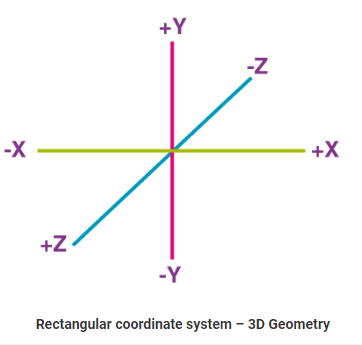There are important formulas related to these topics in NCERT Class 12 Maths Book, which are important to learn.

The use of Three Dimensional Geometry is used to understand various types of shapes and figures.

Important Formulas:-

• Direction cosines of a line joining two points A and B:

(x2 - x1)/AB,(y2 - y1)/AB,(z2 - z1)/AB

where AB = √[(x2 - x1)2 + (y2 - y1)2 + (z2 - z1)2]

• cosθ = |[a1a2 + b1b2 + c1c2] / √(a1 + b1 + c1)2√(a2 + b2 + c2)2]|

where a1, b1, c1 and a2, b2, c2 are direction ratios and θ is the acute angle between the two lines.

11.1 Introduction: Direction cosines and direction ratios of a line joining two points along with the equations of lines and planes in space under different conditions, angle between two lines, two planes, a line and a plane, shortest distance between two skew lines and distance of a point from a plane have been covered in this chapter.

11.2 Direction Cosines and Direction Ratios of a Line: This section enables students to know the definition of Direction Cosines and Direction Ratios of a line as well as how to denote them. Relation between the direction cosines of a line and Direction cosines of a line passing through two points are also covered in this section.

11.3 Equation of a Line in Space: This portion covers the vector and cartesian equations of a line in space. Furthermore, the topic Equation of a line through a given point and parallel to a given vector, Equation of a line passing through two given points, Derivation of cartesian form from vector form have been covered.

11.4 Angle between Two Lines: With the help of this section, a student can learn to find the angle between two lines.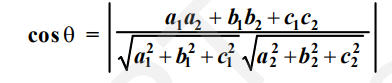11.5 Shortest Distance between Two Lines: Non coplanar or Skew Lines are explained in this section. Subsection 11.5.1 covers the Distance between two skew lines, 11.5.2 covers Distance between parallel lines. These are elaborated in section 11.5 of NCERT Class 12 Maths.

11.6 Plane: There are some terms to determine the plane uniquely. Equation of a plane in normal form, Equation of a plane perpendicular to a given vector and passing through a given point, Equation of a plane passing through three non collinear points, Intercept form of the equation of a plane, Plane passing through the intersection of two given planes are the topics which have been elaborated in this section 11.6.

11.7 Coplanarity of Two Lines: This part of the chapter includes some of the good examples to teach students to show how the lines are coplanar.

11.8 Angle between Two Planes: Section 11.8 defines the angle between two planes by suitable example. One note from this section is, if the planes are parallel, then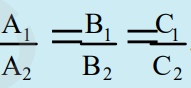11.9 Distance of a Point from a Plane: This section includes various examples to teach students how to find the distance of a point from a plane in two forms: Vector form & Cartesian Form.

11.10 Angle between a Line and a Plane: In this section, the statement is given: The angle between a line and a plane is the complement of the angle between the line and normal to the plane. This statement has been proved using the vector form.

Study Tip for Three – Dimensional Geometry (3D):-Practice eas questions time and again, many find it difficult to comprehend so, intense practice is important. Developing a deep understanding in the concepts and formulas are useful to answer all the questions of 3D.

#### Chapter 12:- Linear Programming

Linear programming is one of the best industry standard mathematical processes to find out the best level of production. According to mathsisfun, maybe planning was a closer word to be chosen when computer language wasn’t very common.

The basic application of linear programming is to maximise and minimise the numerical value. It is used on a large scale in economics, business, telecommunication, and manufacturing fields.

This chapter helps you to learn the related terminology, objective function, types of linear programming (L.P.), etc.

12.1 Introduction: Linear programming can be solved using various methods, however, the chapter 12 of NCERT class 12 Maths helps students to learn linear programming problems and their solutions by graphical method only.

12.2 Linear Programming Problem and its Mathematical Formulation: This is an important section of the chapter 12.2 as it helps students to learn various important points: constraints, Optimisation problem, objective function, decision variables, Feasible region, infeasible solution, etc. Along with these terms, two theorems have been covered.

12.3 Different Types of Linear Programming Problems:  There are three linear programming problems that are covered in this section: Manufacturing Problems, Diet Problems, Transportation Problems.

Study Tip for Linear Programming:- In prerequisites you should have a good common on equations and basic mathematical calculations. With lots of practice, it should not be challenging to score good marks in this topic.

#### Chapter 13:- Probability

Class 12 Maths Chapter 13 Probability is not a simple chapter like others in previous standard. Class 12 Probability is a bit advanced topic, in this lesson you will find various theorems such as Bayes’ theorem, binomial distribution, multiplication theorem on probability, independent events etc.

Students referring to the Class 12 NCERT Maths Book, will find that this lesson focuses on conditional probability.

As per the definition 1 of NCERT book, If E and F are two events connected with the same sample space of a random experiment, the conditional probability of the event E given that F has occurred, i.e. P (E|F) is given by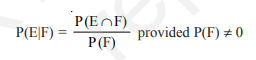13.1 Introduction: The intro section informs students what topics they are about to study in chapter 12 probability. The Concepts of Conditional Probability, Baye's Theorem, Multiplication rule of probability and independence events as well as various important concepts are discussed in this chapter.

13.2 Conditional Probability: The definition of conditional probability as well as their properties have been covered in section 13.3.

13.3 Multiplication Theorem on Probability: Multiplication rules of probability have been wrapped in this section along with the Multiplication rule of probability for more than two events.

13.4 Independent Events: This section is fully committed to teach students the definition of Independent Events. There are several related examples and problems have been mentioned.

13.5 Bayes' Theorem: The Baye’s Theorem has been proved in this section. Bayes Theorem is a mathematical formula for determining the Conditional Probability.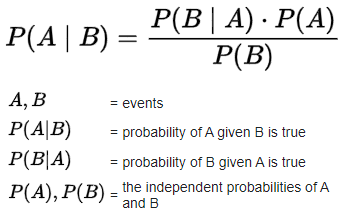13.6 Random Variables and its Probability Distributions: About random experiments, sample spaces and such more related topics are discussed in this section 13.6. The section has been divided into various subtopics: Probability distribution of a random variable, Mean of a random variable, and Variance of a random variable,

13.7 Bernoulli Trials and Binomial Distribution: When independent trials have only two outcomes are called Bernoulli Trials. This trial has been briefly explained in section 13.7. Also Binomial distribution has been covered.

Study Tip for Probability:- You must have a deeper understanding in basic level probability to be able to answer class 12 probability. Focus on understanding the theorems and practice a bunch of questions on them.

### Importance of Class 12 Maths NCERT Solutions

Class 12 Maths NCERT Solutions play a significant role in student's learning path as it helps them to identify what wrong or right approaches they have implemented while solving the maths questions.

By taking a look at the given advantages a student can know Importance of Class 12 Maths NCERT Solutions:

• It Develops the Conceptual Understanding:-Conceptual understanding is the core of learning, it is vital to have a clear understanding in the topics you study. Because as you develop the Conceptual Understanding, it will help you face any kind of challenging questions. The NCERT Class 12 Maths Solutions is the source that can aid you to learn and develop the fundamental knowledge not to ace the board questions but all kinds of advanced level problems.
• It not only helps in comprehending the topics and concepts easier but helps them to reduce their time spent on maths questions while revising.Eventually, Referring to the Maths Solutions of class 12 helps students to stay ahead in academics of their fellow students. Therefore, Class 12 Maths NCERT Solutions are considered Important - it helps a lot in tackling the competition.
• Helps In Preparing for the Exam:-Maths experts develop the NCERT 12 Maths Solutions according to the syllabus and exam pattern. Therefore, using the solutions has advantages. It allows board candidates to understand the right method of writing their board papers of mathematics.
• To Cross-Check Answers & Revise Topics:-Cross checking of an answer is vital as it helps in identifying the mistakes. Cross checking also allows learners to know what could be the right method of solving a particular topic. In the examination hall or while making the notes, students are needed to maintain the higher accuracy. So, NCERT Maths 12th Solutions helps in doing so.

### Why SelfStudys NCERT Solutions for Class 12 Maths is a Good Choice?

• Step Wise Solutions written by subject experts for all 13 chapters.
• These solutions have very precise and easy to understand answers. Thus it is easy to understand.
• The answer book is one of the best study materials that boost the student’s confidence.
• Since the questions are easier to understand, it is easier to score higher in the board exams.
• Our mathematics experts have followed the exam pattern as well as syllabus to make the Class 12 Maths NCERT Solutions in accordance with the requirements of the board examination.

### Question Paper Design Of Class 12 (2021-2022) - Mathematics

To have a perfect exam preparation of class 12 mathematics, you must have a good knowledge of the question papers design 2021-2022 of the maths subject. CBSE Class 12 Mathematics Sample Papers can help you to be familiarise with the question paper design.

We at Selfstudys, provide sample papers and previous year question papers to help you to be familiar with the question paper design.

Time: 3 hours Max. Marks: 80

 Sr No. Typology of Questions Total Marks % Weightage 1 Remembering: Exhibit memory of previously learned material by recalling facts, terms, basic concepts, and answers. Understanding: Demonstrate understanding of facts and ideas by organizing, comparing, translating, interpreting, giving descriptions, and stating main ideas 44 55 2 Applying: Solve problems to new situations by applying acquired knowledge, facts, techniques and rules in a different way. 20 25 3 Analysing : Examine and break information into parts by identifying motives or causes. Make inferences and find evidence to support generalizations Evaluating: Present and defend opinions by making judgments about information, the validity of ideas, or quality of work based on a set of criteria. Creating: Compile information together in a different way by combining elements in a new pattern or proposing alternative solutions 16 20 Total 80 100
 INTERNAL ASSESSMENT 20 MARKS Periodic Tests ( Best 2 out of 3 tests conducted) 10 Marks Mathematics Activities 10 Marks

#### Detailed Exam Pattern of CBSE Class 12 Maths

As per the latest Syllabus of CBSE Class 12 Mathematics, the mathematics subject has assigned 20 marks for project work, whilst 80 marks for theory sections or written examination out of 100 marks.

The detailed exam pattern of CBSE Class 12 Maths are as follows:-

1. Calculus has a higher weightage of 44 marks, thus students must pay attention to each topic that comes under the calculus. To solve questions of calculus a student must have a good logical approach, which can be acquired by thorough practice of each question.
2. Two chapters: Vectors and Three Dimensional Geometry contain 17 marks weightage. Scoring full marks from these chapters is a bit easier, if you do rigorous practice and make diagrams, as it helps in better visualisation.
3. Algebra and Probability sections are important and easier. So, to score better from these topics, one must have their basics clear.
4. Chapter 1 - Relations and Functions, and Chapter 12- Linear Programming are easier than other lessons in Class 12 maths, so weekly/monthly revision of these chapters are more than enough to score decent marks in these topics.
5. Mathematics subjects require a more logical approach than theory based or formulas based approach. So, keep in mind to complete 6 units of NCERT Class 12 Maths to be able to score full marks in the board examination.

### How are NCERT Class 12 Maths Solutions Helpful For Board Exams?

NCERT Class 12 Maths Solutions is very helpful to practice the questions and to understand them. Students aiming to score above 90% marks in their 12th board exams can refer to this NCERT Solutions, which will enable them to clear their doubts and confusion easily.

By doing regular revision and numerous practice questions, students can master their 12th mathematics and score very high marks in the board examination.

It is believed that by solving questions with the help of Class 12 Maths NCERT Solutions, students develop good problem solving skills.

NCERT Exemplar for Class 12 MathsNCERT Exemplar Class 12 Maths Solutions is a bit advanced in terms of questions level. Each chapter of class 12th mathematics exemplar consists of questions that challenge students’ learning of maths. Exemplar questions are developed keeping in mind the syllabus, it also enables candidates to practice more questions so that they can easily crack the board exams and competition level of exams.

NCERT Class 12th maths contains an equal number of chapters as available in the NCERT Exemplar Class 12 Maths textbooks. However, with the help of exemplar learners can have access to the quality problems. Those problems not only help in boosting the scores but make students more creative and analytical thinkers.

#### Unit wise weightage of CBSE Class 12CBSE Class 12th Mathematics has a total of 13 chapters out of which 7 chapters for term 1 exam and 8 chapters for term 2 exams. A good understanding of these chapters is important but knowing about their weightage is also crucial. Therefore, here we have provided the unit wise weightage for both the term examination. Go through the table to understand the marks weightage of each chapter of mathematics.

#### Unit wise weightage of CBSE Class 12 First TermIn term 1 exam, questions were asked from 7 chapters all those chapters were taken from 5 units. Below you can check their unit names and mark weightage of those units.

 Unit Unit Name Marks 1 Relations and Functions 08 2 Algebra 10 3 Calculus 17 5 Linear Programming 05 Total 40 Internal Assessment Marks 10 Total Marks 50

#### Unit wise weightage of CBSE Class 12 Second TermCalculus, Vectors, 3D, and Probability are the three units for term 2 exams. Questions in the 2nd term examination of CBSE Class 12th will be asked from these units. You can be thorough with their marks weightage from the below-given table.

 Unit Unit Name Marks 3 Calculus 18 4 Vectors and Three-Dimensional Geometry 14 6 Probability 08 Total 40 Internal Assessment Marks 10 Total Marks 50

#### How To Solve NCERT Maths Questions With Ease?Including 12 Class Maths Chapters plus NCERT Exemplar Class 12 Maths 13 chapters become a total of 26 chapters to solve.

Solving these 26 chapters is not only important but it is the best to gather a deep knowledge of the advanced level of maths questions.

Since these questions of class 12 maths are of advanced level, students may find it difficult to answer. Therefore, here are the 3 simple yet most effective ways to solve NCERT Maths Questions With Ease:

• Understand the Question Type

Direct numerical maths questions, Word Problems, and combined types of questions are available in class 12 mathematics.

Therefore, firstly understand the questions types and try your best to answer them by using the appropriate formulas, law,s or concepts you have studied in NCERT Maths Books.

Having a good understanding of the questions types can ease your mind to initiate the process of finding the right methods to answer them.

• Solve Them In Simple Steps

Math questions are solved ideally by breaking them down into simple steps. Doing this helps in a better understanding of the problem-solving approach.

It also assists students to plan the next steps they should take to reach accurate answers.

• Use NCERT Solutions, If Unable To Solve Maths Questions

Even after applying the above two tips, you might still face difficulty, but using Class 12 Maths NCERT Solutions will surely help you to answer the questions with ease.

Because it is prepared by the experts who have broken down the questions and their answers into simple steps by applying the appropriate formula, laws, and concepts.

#### FAQ. On NCERT Solutions For Class 12 MathsFAQs

There are a total of 13 Chapters in NCERT Solutions For Class 12 Maths. Both problems and their detailed solutions are provided in the Selfstudys NCERT Solutions for class 12th.

Getting a good score or high score in class 12th board exams are crucial for the students, because it plays an essential role to the students future career. Therefore, Solving NCERT Solutions for class 12 maths regularly is important. The solutions make sure that there is no doubt left to the students.

You can download the NCERT Solutions for class 12 maths from the Selfstudys website. These solutions are prepared by subject matter experts that ensure to give you a complete knowledge about the chapters and topics that are mentioned in the NCERT Class 12th Mathematics books. The solutions are available for free to download.

Yes, it is easier than class 11 maths. However, to answer the class 12th maths you must have a strong grip on basic and fundamentals of mathematics.

Yes, class 12 NCERT Maths is enough for the CBSE Board exams because the NCERT Class 12 Maths Books are prescribed by the CBSE board.

The best website for NCERT Solutions of class 12 maths is Selfstudys, because the solutions are prepared by our subject matter experts.

Very simple: Practice maths questions from NCERT Maths books, Previous Year Question Papers, and Sample Question Papers. You will be able to develop a good understanding of the exam pattern and the types of questions that are asked in the board exams. It will help you to score 90 or even more than that.

Chapters that come under Calculus are considered as tough chapters in Class 12 Maths. However, you can master this topic if you revise them multiple times and solve relevant questions often.

The very first thing to keep in mind is to pay attention to the prescribed syllabus. Go through all the chapters and solutions to have a better understanding of the subject. Then refer to the question papers to practice the actual board-level problems and solve them as much as possible.

The time you enjoy studying is the best time to study maths. Although, many students think they are more productive and focused in the nighttime. Therefore, try studying both day and night and check what time suits you better.

Yes, NCERT Maths textbooks are important for class 12 boards.

If you want to solve all the mathematics questions given in class 12 maths with ease then yes, NCERT Solution is enough for class 12 maths exam.

Yes, NCERT books are the officially prescribed textbooks and the syllabus is also followed by the NCERT Books and solutions so, yes all questions on board come from Class 12 Maths NCERT Solutions.

13 Chapters are there in NCERT 12 Maths.

None.

Related News
See All×
Open Now